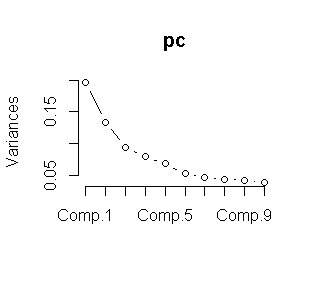Want to share your content on R-bloggers? click here if you have a blog, or here if you don't.

The following R-script is for download the data automatically from twitter using the package `SchedulerR`. As first step we download the data using the `OAuth protocol` and store the data using as a name the date from the download. As second we used the package `SchedulerR` for to specify how long the script runs. This package has the advantage that we can set the time and also how long will repeat either daily, weekly or monthly.

```library(twitteR)
library(httr)
library(SchedulerR) ```

Also we choose the directory where the RData would be saved:

`setwd("C:/Users/Documents/schedulerR")`

OAuth protocol:

```api_key <- "xxxxxxxxxxxxxxxxxxxxxx"
api_secret <- "xxxxxxxxxxxxxxxxxxxxxxxxxxxxxxxxxx"
access_token <- "xxxxxxxxxxxxxxxxxxxxxxxxxxxxxxxx"
access_token_secret <- "xxxxxxxxxxxxxxxxxxxxxxxxxxxxxxxxxxxxxx"

Extract the tweets using usertimeline function from the Twitter R package:

``` clinton_tweets <- userTimeline("HillaryClinton", n = 3200)
tweetsc.df <- twListToDF(clinton_tweets)
dim(tweetsc.df)```

Create a variable called date and become to character:

```date<-Sys.Date()
date<-as.character(date)
name<-paste(date,".RData")
#Finally we save the Rdata using as name the date from the download
save(tweetsc.df, file =name)```

The next step is used the package SchedulerR, the script for this is the following:

```taskscheduler_create(taskname = "taskclinton", rscript = clintontweets,
schedule = "DAILY", starttime = "11:30", startdate = format(Sys.Date(), "%d/%m/%Y")) ```

## Examples of Analysis

We can implement many analysis from text data.

Find words common with a minimun frequency of 45:

```term.freq <- rowSums(as.matrix(tdm))
term.freq = 45)
#we saved the data in a data frame
df <- data.frame(term = names(term.freq), freq = term.freq)
save(df,file="tqfclinton50.RData")
#plot with ggplot2
library(ggplot2)
ggplot(df, aes(x = term, y = freq)) + geom_bar(stat = "identity") +
xlab("Terms") + ylab("Count") + coord_flip()```

Hierarchical cluster:

```# remove the parse terms
tdm2 <- removeSparseTerms(tdm, sparse = 0.95)
m2 <- as.matrix(tdm2)
# hierarchical cluster
m <- as.matrix(m2)
d<- dist(m2)
#we used the cosine similaruty as distance because is the best option for text data
distcos<-dissimilarity(x=m2,method='cosine')
groups <- hclust(distcos,method="complete")
#plot dendograma
plot(groups, hang=-1)
rect.hclust(groups,5)```

### K means clustering

We combine the Kmeans clustering with Principal components analysis for obtain a better result. As a first we used the elbow method for choose the “K”We choose K=5 from the plot. Now we proceed to make the K means clustering.

```pc <- princomp(tdm)
plot(pc)
data<-tdm
wss <- (nrow(data)-1)*sum(apply(data,2,var))
for (i in 2:15) wss[i] <- sum(kmeans(data,
centers=i)\$withinss)
plot(1:15, wss, type="b", xlab="Number of Clusters",
ylab="Within groups sum of squares")

m3 <- t(tdm2) # transpse from term document matriz
set.seed(1222) # we choose a seed.
k <- 5# number of clusters
kmeansResult <- kmeans(m3, k)
km<-round(kmeansResult\$centers, digits = 3)
#we saved in a csv file.
write.csv(km,file="kmeansclinton.csv")```

The following loop is for visualize the clusters.

```for (i in 1:k) {
cat(paste("cluster ", i, ": ", sep = ""))
s <- sort(kmeansResult\$centers[i, ], decreasing = T)
cat(names(s)[1:5], "\n")
}
write.csv(s,file="clusterkm.csv")  ```From the results we can said from each cluster they are the topics most spoken from the tweets.

Related Post